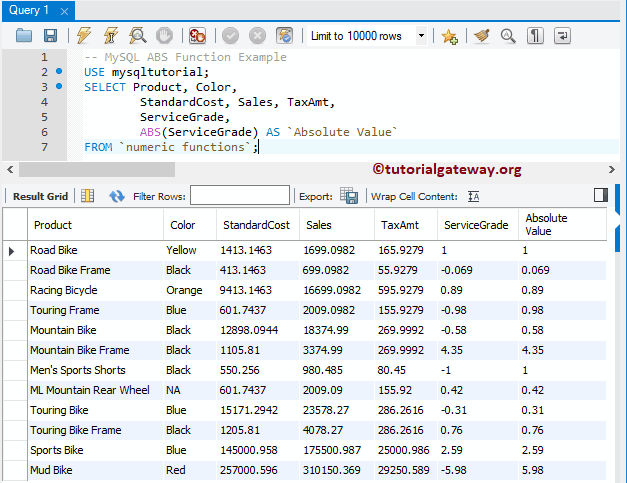# MySQL ABS Function

MySQL ABS function is one of the Numeric Functions, which is beneficial to return the absolute positive value of the specified expression. In this article, we show how to find Absolute Values using Command Prompt and Workbench with an example.

The basic syntax of the ABS in MySQL is as shown below:

```SELECT ABS (Numeric_Expression)
FROM Source```

To demonstrate this Numeric function, we are going to use the below shown data

## MySQL ABS Function Example

It returns the positive value of any numeric. The following query shows multiple ways to use this.

```-- Numeric Value
SELECT ABS(-52) AS `AbsoluteValue`;

-- of Numeric Expression
SELECT ABS(10 + 35 - 102) AS `AbsoluteValue`;

-- of string number
SELECT ABS('-10.02') AS `AbsoluteValue`;

-- of String text
SELECT ABS('MySQL') AS `AbsoluteValue`;```

In the below statement, We used this to find the absolute value of different values. Here, we assigned a new name to the result as ‘Absolute Value’ using the ALIAS Column in MySQL.

The MySQL ABS Numeric Function also allows you to find the absolute value of the data in a column. In this example, we are going to find the absolute numbers for all the records present in the ServiceGrade column.

```SELECT Product, Color,
StandardCost, Sales, TaxAmt,